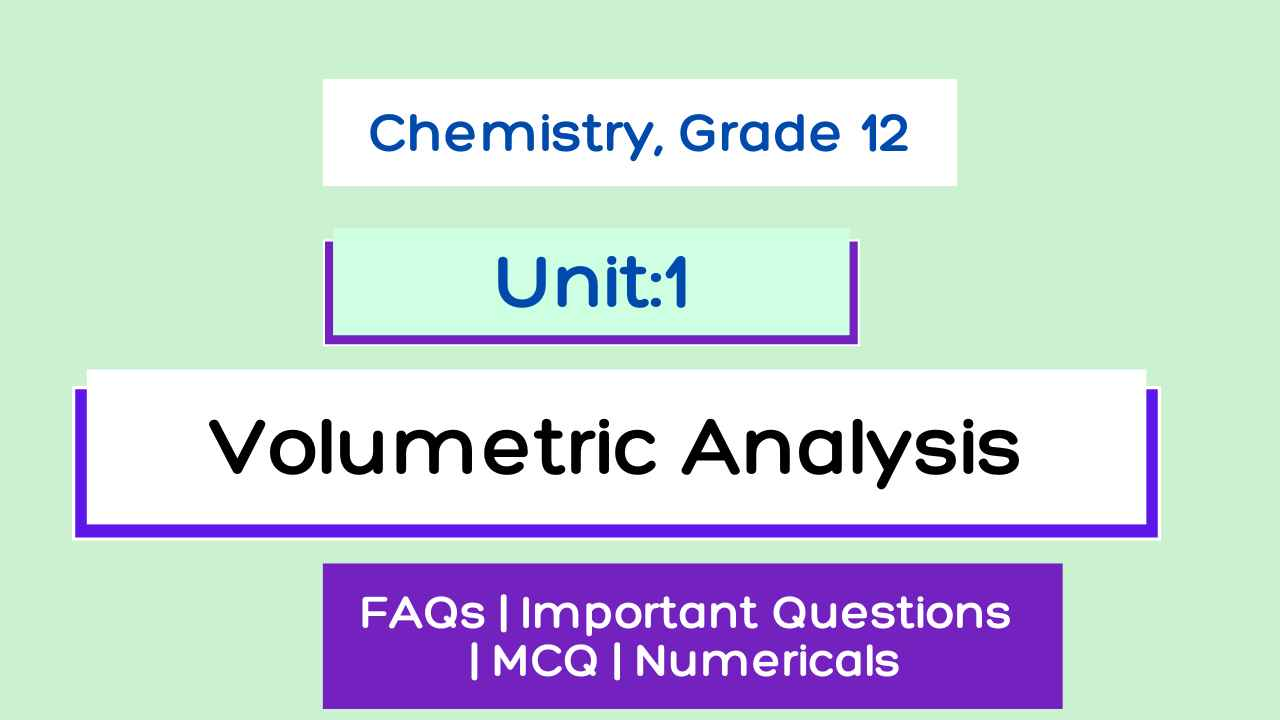Welcome to Narayannepal.com.np

# Volumetric Analysis : Class 12 Physical Chemistry Notes

Volumetric Analysis Class 12 Notes | Unit 1 Chemistry | Pdf Solution | Important Questions | Numericals | Chemistry Class 12 Notes Nepal | NEB Notes |

Unit 1: Volumetric Analysis

Class 12 Chemistry Volumetric Analysis Notes

Volumetric analysis Course content
1.1 Define and explain the terms volumetric and gravimetric analysis.
1.2 Express the concentration of solutions in terms of percentage, g/l, molarity, molality, normality, ppm, ppb
1.3 Define and calculate the equivalent weight of (elements, acids, bases, salts, oxidizing and reducing agents).
1.4 Express the concentration of the solution in terms of normality.
1.5 Explain and apply the concept of the law of equivalence in chemical calculation.
1.6 Define and explain primary and secondary standard substances.
1.7 Explain different types of titration and their applications.Unit 1: Volumetric Analysis

## Volumetric analysis Introduction

Volumetric analysis is defined as the process of the determination of the concentration of an unknown solution by finding its volume which will exactly react with a definite volume of another solution of known concentration.

Gravimetric analysis is defined as the process of quantitative determination of an analyte(the ion being analyzed) based on the mass of a solid.

At first solution of given sample is prepared, treated with suitable reagent to convert into insoluble form which is made pure by washing, dried and weighed. Then the weight of the required substance is calculated with the help of theoretical concept.

Eg. How much SO4-- ion is present in a given sample of Na2SO4?

A known amount of the given sample is dissolved in water and the excess amount of BaCl2 is added to the solution so that all SO4-- is converted into insoluble BaSO4. The precipitate is washed and made pure, it is then dried and weighed. Let the weight of BaSO4 formed is Y gm.

We know, 233 gm. of BaSO4 contains 96 gm. of SO4-- ions.
So, Y gm. of BaSO4 contains (96/233) x Y gm. of SO4--ions.

Pre-requirements in volumetric analysis

Equivalent weight (equivalent mass)

It is defined as “the no. of parts by wt. of the substance that combines with or displaces 1.008 parts by wt. of hydrogen, 8 parts by wt. of oxygen or 35.5 parts by wt. of chlorine.”

Equivalent weight is a unit less.

Equivalent weight expressed in gm is called gm. equivalent.

No. of gm. eqvt. = given wt./eq.wt.

## Volumetric Analysis Complete Notes - NEB Class 12 Chemistry

Volumetric analysis
Gravimetric analysis

Eg. How much SO4 -- ion is present in a given sample of Na2SO4?

### Pre-requirements in volumetric analysis

Equivalent weight (equivalent mass)

1. Primary standard solution:
2. Secondary standard solution:
Volumetric Analysis Notes Pdf

This is a Pdf with volumetric analysis notes.

Queries Solved in this Volumetric Analysis Notes:

1. Volumetric Analysis Class 12 Notes
2. Volumetric Analysis Notes Pdf
3. Volumetric Analysis Class 12 Important Questions
4. Volumetric Analysis Numericals
5. Class 12 Chemistry
6. Volumetric analysis Class 12 Exercise Solutions
Getting Info...